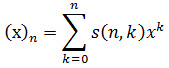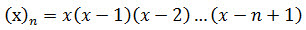# SQL Server Stirling Number of the First Kind function

STIRLING1
Updated: 21 July 2017

Use the SQL Server scalar function STIRLING1 to calculate the Stirling numbers of the first kind. The Stirling numbers of the first kind are the coefficients in the expansion:Where (x)n is the falling factorial (see FACTORIALPOWER).Stirling numbers of the first kind exhibit the following recursive relationship.Syntax
SELECT [wct].[STIRLING1] (
<@n, int,>
,<@k, int,>)
Arguments
 Input Name Definition @n Number of items @k Number chosen
Return Type
float
Remarks
• If @n <= 0 then NULL is returned.
• If @k < 0 then NULL is returned.
• If @k > n then NULL is returned.
• s(0,0) = 1
• s(n,0) = 0
• Available in XLeratorDB / statistics 2008 only
Examples
Example #1

Calculate the coefficients for the expansion of: x(x-1)(x-2)(x-3)(x-4)

SELECT
wct.STIRLING1(4,1) as [x]
,wct.STIRLING1(4,2) as [x^2]
,wct.STIRLING1(4,3) as [x^3]
,wct.STIRLING1(4,4) as [x^4]

This produces the following result.

The expanded equation would thus be: x4-6x3+11x2-6x

Example #2

A lower triangular representation of the first few Stirling numbers of the first kind.

SELECT ,,,,,,,,,
FROM (
SELECT
n.SeriesValue as n
,k.seriesValue as k
,cast(wct.STIRLING1(n.SeriesValue,k.SeriesValue) as int) as S1
FROM
wct.SeriesInt(1,10,NULL,NULL,NULL)n
CROSS APPLY
wct.SeriesInt(1,n.seriesvalue,NULL,NULL,NULL)k
)d
PIVOT (MAX(S1) FOR k IN (,,,,,,,,,))pvt
ORDER BY
n

This produces the following result.Copyright 2008-2021 Westclintech LLC         Privacy Policy        Terms of Service